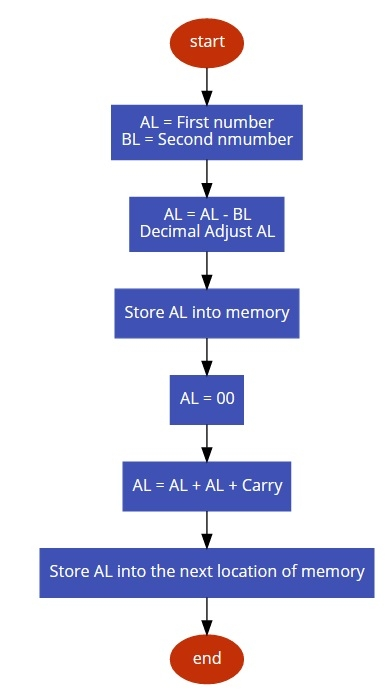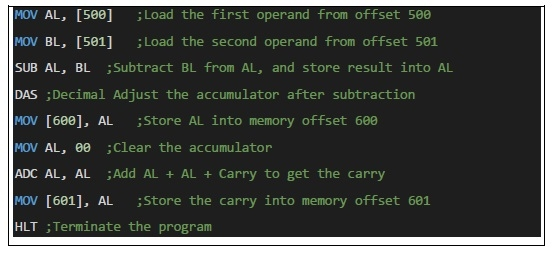# 8086 program to subtract two 8 bit BCD numbers

In this program we will see how to subtract two 8-bit BCD numbers.

## Problem Statement

Write 8086 Assembly language program to subtract two 8-bit BCD number stored in memory address offset 600.

## Discussion

This task is too simple. Here we are taking the numbers from memory and after adding we need to put DAS instruction to adjust the accumulator content to decimal form after the subtraction operation. The DAS will check the AC and CY flags to adjust a number to its decimal form.

Data

500
99
501
25

## Flow Diagram## Program